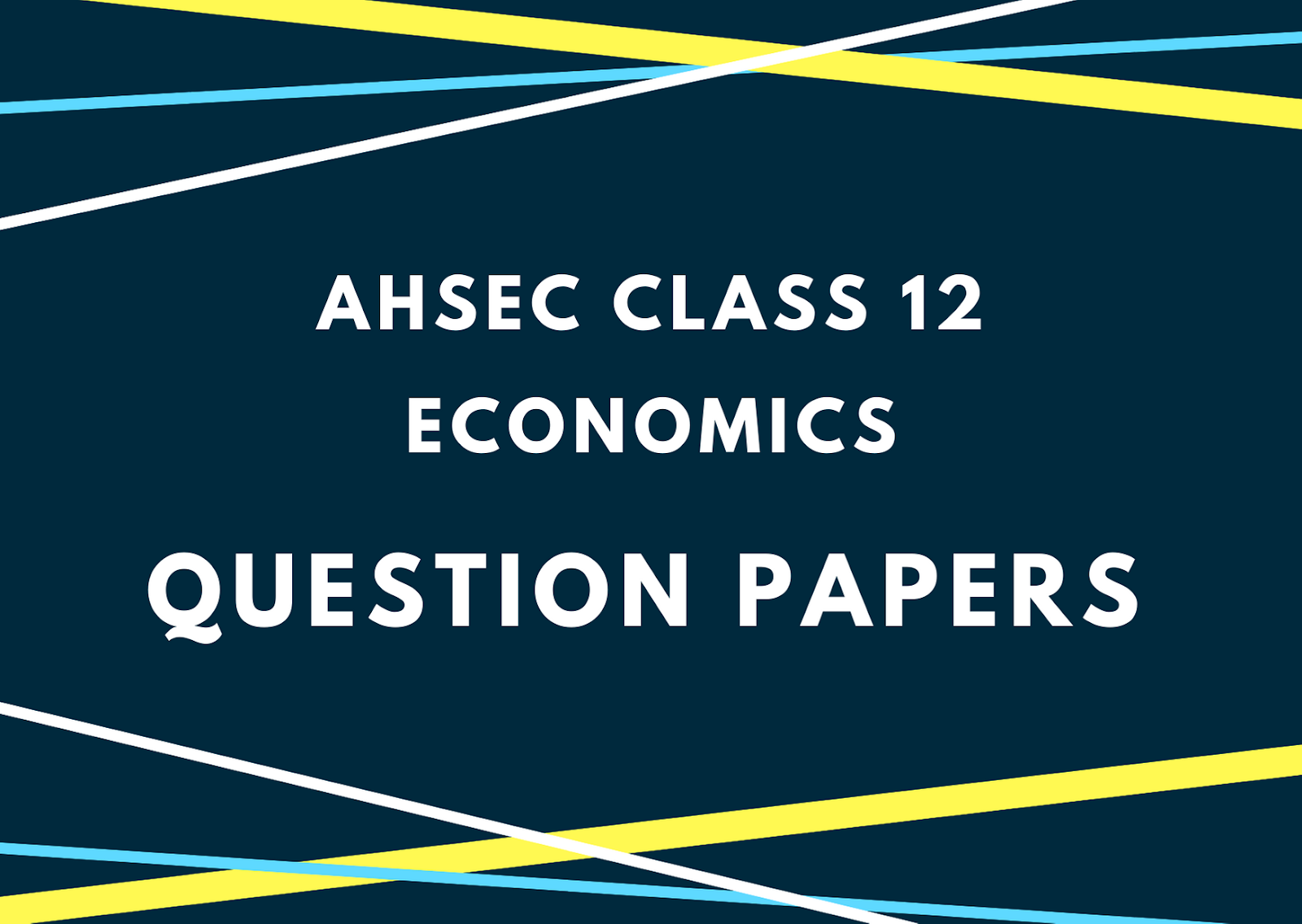# AHSEC Class 12 Economics Question Paper 2013## AHSEC Class 12 Economics Question Papers 2013ACCOUNTANCYFull Marks: 100Pass Marks: 30Time: Three HoursFull Marks: 100 Time: 3 hour
1. (a) What is mixed economy? 1
(b) Give one example of complementary goods. 1
(c) If the percentage change in quantity demanded is equal to the percentage change in price, what is the price elasticity of demand? 1
(d) Define average variable cost. 1
(e) Fill up the blank:
For a firm under perfect competition, the average revenue = ____. 1
(f) What does a vertical supply curve imply? 1
2. Give one example of each of goods and services. 2
3. Explain how price elasticity of demand for a good depends on its nature. 2
4. Why does an indifference curve slop downward? 2
5. State the law of variable proportions. 2

6. State the concept of utility function. 2
7. What is shut down point of a firm? 2
8. The monthly income of a consumer is Rs. 400 and he spends this income entirely on two commodities, X and Y. The Price of the commodity X is Rs. 20 and that of Y is Rs. 25. On the basis of this information:
1. Draw the budget line of the consumer.
2. What will happen to the budget line if the price of commodity Y decreases to Rs. 20 while the income of the consumer and the price of commodity X remain the same? 2+2=4
9. What is budget line? Why does it slope downward? 1+3=4
10. What is an Isoquants? Explain it graphically. 1+3=4
11. The production function of a firm is given as. Calculate the level of output when it employs 16 units of labourand 36 units of Capital. 4
12. Show that the average fixed cost curve is a rectangular hyperbola. 4
13. Show how the price elasticity of demand is graphically measured along a linear demand curve. 6
Or
Prove that at consumer’s optimum point on an indifference curve, the marginal rate of substitution is equal to the ratio of the prices.
14. “Monopoly firm is a price maker” – Explain. 6
Or
The demand and supply functions of a firm under perfectly competitive market are given below:AndFind (i) The equilibrium level of output and price.
(ii) If due to increased cost, the supply function becomes, what will be the changes in equilibrium price and quantity?15. (a) What is cash reserve ratio? 1

(b) Define high-powered money. 1
(c) Choose the correct one and fill in the gap:
The ratio of additional consumption to additional income is called ____. (marginal propensity to save/marginal propensity to consume/marginal income). 1
(d) What is aggregate supply? 1
(e) What is balance of payments? 1
(f) What is value added of a firm? 1
16. What is national disposable income? 2
17. What is net national product at factor cost? 2
18. Give the concept of transfer payment along with an example. 2
19. In an open economy, if the marginal propensity to consume (c) is 0.8 and the marginal propensity to import (m) is 0.2, find the size of the income multiplier. 2
20. What is inflation? 2
21. What is ex-ante investment? 2
22. A firm produces goods of Rs. 500 per year and intermediate goods used by the firm is of worth Rs. 250. The cost of capital consumption is Rs. 20 per year. Calculate gross value added and net value added by the firm. 4
23. Briefly explain the components of aggregate demand. 4
24. Distinguish between autonomous investment and induced investment. 4
25. Trace out the relationship between government deficit and government debt. 4
26. What are the basic objectives of a government budget? – Explain them briefly. 4
27. Discuss the reasons why it will not be correct to say that the GDP is the index for measuring welfare of the people of a country. 6
Or
Explain the income method of calculating GDP.
28. Explain the role of the RBI as the lender of the last resort. 6
Or

Explain the concept of deficit financing.